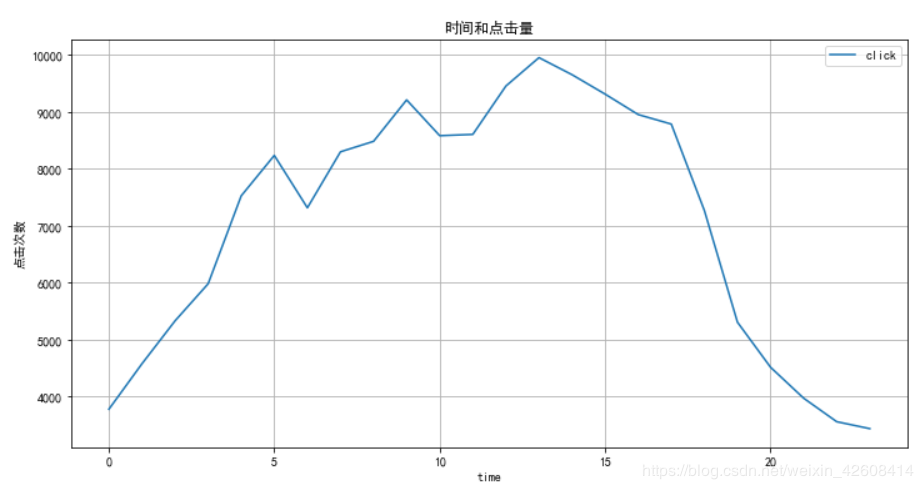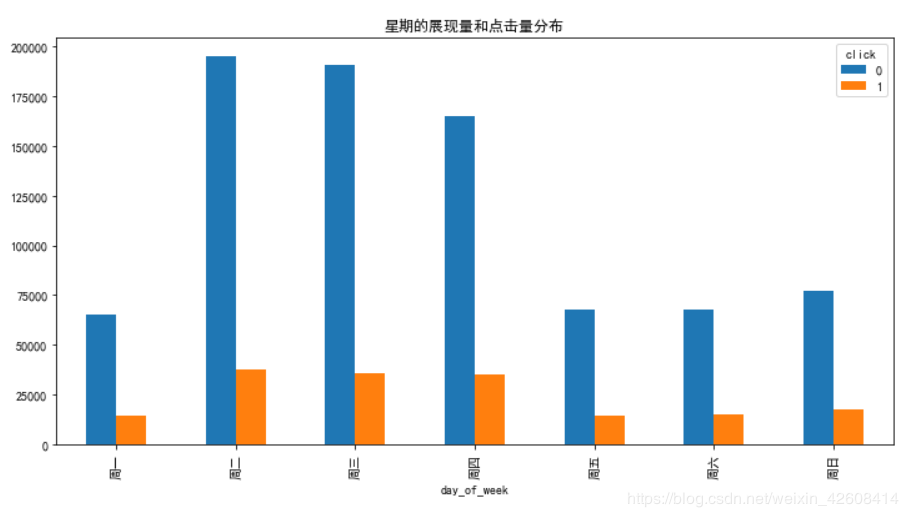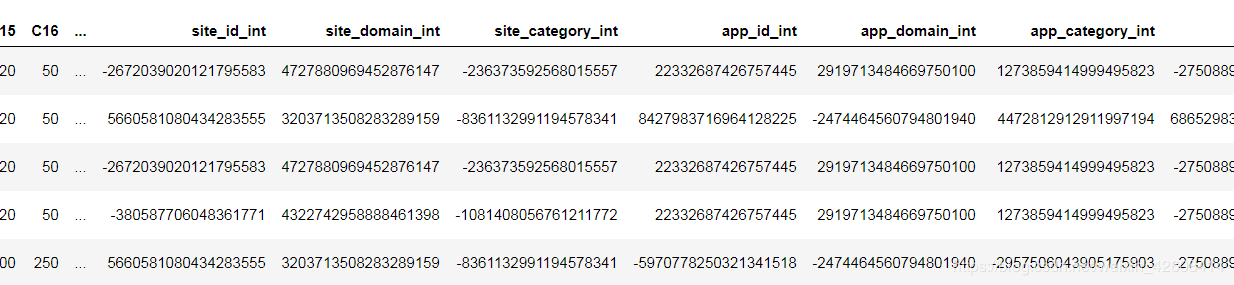# 使用python进行广告点击率的预测的实现

更新时间：2019年07月04日 09:38:16   转载 作者：-派神-

```types_train = {
'id': np.dtype(int),
'click': np.dtype(int),    #是否点击,1表示被点击,0表示没被点击
'hour': np.dtype(int),    #广告被展现的日期+时间
'C1': np.dtype(int),     #匿名分类变量
'banner_pos': np.dtype(int), #广告位置
'site_id': np.dtype(str),   #站点Id
'site_domain': np.dtype(str), #站点域名
'site_category': np.dtype(str), #站点分类
'app_id': np.dtype(str),    # appId
'app_domain': np.dtype(str),  # app域名
'app_category': np.dtype(str), # app分类
'device_id': np.dtype(str),   #设备Id
'device_ip': np.dtype(str),   #设备Ip
'device_model': np.dtype(str), #设备型号
'device_type': np.dtype(int),  #设备型号
'device_conn_type': np.dtype(int),
'C14': np.dtype(int),  #匿名分类变量
'C15': np.dtype(int),  #匿名分类变量
'C16': np.dtype(int),  #匿名分类变量
'C17': np.dtype(int),  #匿名分类变量
'C18': np.dtype(int),  #匿名分类变量
'C19': np.dtype(int),  #匿名分类变量
'C20': np.dtype(int),  #匿名分类变量
'C21':np.dtype(int)  #匿名分类变量
}

n = 40428967 #数据集中的记录总数
sample_size = 1000000
skip_values = sorted(random.sample(range(1,n), n-sample_size))
parse_date = lambda val : pd.datetime.strptime(val, '%y%m%d%H')

with gzip.open('./data/ctr/train.gz') as f:
train = pd.read_csv(f, parse_dates = ['hour'], date_parser = parse_date, dtype=types_train, skiprows = skip_values)
print(len(train))```print(train['click'].value_counts())
print()
print(train['click'].value_counts()/len(train))

sns.countplot(x='click',data=train, palette='hls')
plt.show()```在“click”变量的统计数据中，点击的数量大约占17%，未点击的数量大约占83%。也就是说广告的平均点击率大概是在17%左右。

```print(train.hour.describe())

train.groupby('hour').agg({'click':'sum'}).plot(figsize=(12,6))
plt.ylabel('点击量')
plt.title('时间和点击量')
``````train['time'] = train.hour.apply(lambda x: x.hour)
train.sample(5)``````train.groupby('time').agg({'click':'sum'}).plot(figsize=(12,6),grid=True)
plt.ylabel('点击次数')
plt.title('时间和点击量')
``````train.groupby(['time', 'click']).size().unstack().plot(kind='bar', figsize=(12,6))
plt.ylabel('数量')
plt.title('展现量与点击量');```我们将时间按每个时间点展开,这里没有特别之处下午1点的展现量最大,所以点击量也是最大,我们发现展现量和点击量似乎是成正比的。这似乎也告诉我们，如果您要投放在线广告，请在下午1点至2点之间投放,因为此时广告的展现量和点击量都是最大的。

```df_click = train[train['click'] == 1]
df_hour = train[['time','click']].groupby(['time']).count().reset_index()
df_hour = df_hour.rename(columns={'click': 'impressions'})
df_hour['clicks'] = df_click[['time','click']].groupby(['time']).count().reset_index()['click']
df_hour['CTR'] = df_hour['clicks']/df_hour['impressions']*100```plt.figure(figsize=(12,6))
sns.barplot(y='CTR', x='time', data=df_hour)
plt.title('点击率的时间分布')``````train['day_of_week'] = train['hour'].apply(lambda val: val.weekday_name)
cats = ['Monday', 'Tuesday', 'Wednesday', 'Thursday', 'Friday', 'Saturday', 'Sunday']
train.groupby('day_of_week').agg({'click':'sum'}).reindex(cats).plot(figsize=(12,6))
ticks = list(range(0, 7, 1))
labels = "周一 周二 周三 周四 周五 周六 周日".split()
plt.xticks(ticks, labels)
plt.title('星期的点击量')``````train.groupby(['day_of_week','click']).size().unstack().reindex(cats).plot(kind='bar', title="Day of the Week", figsize=(12,6))
ticks = list(range(0, 7, 1))
labels = "周一 周二 周三 周四 周五 周六 周日".split()
plt.xticks(ticks, labels)
plt.title('星期的展现量和点击量分布')```从上图可知星期二的展现量和点击量是最高的，接下来是星期三和星期四，不过展现量和点击量较高并不意味着点击率也较高，因此接下来我们要按星期来计算一下点击率。

```df_click = train[train['click'] == 1]
df_dayofweek = train[['day_of_week','click']].groupby(['day_of_week']).count().reset_index()
df_dayofweek = df_dayofweek.rename(columns={'click': 'impressions'})
df_dayofweek['clicks'] = df_click[['day_of_week','click']].groupby(['day_of_week']).count().reset_index()['click']
df_dayofweek['CTR'] = df_dayofweek['clicks']/df_dayofweek['impressions']*100

plt.figure(figsize=(12,6))
sns.barplot(y='CTR', x='day_of_week', data=df_dayofweek, order=['Monday', 'Tuesday', 'Wednesday', 'Thursday', 'Friday', 'Saturday', 'Sunday'])
plt.title('星期的点击率')```C1是一个匿名的分类型变量，我们不知道它的含义，我们先查看一下c1的数据分布

`print(train.C1.value_counts()/len(train))`因为C1是分类型变量，它的值包含了1005,1002,1010,1012,1007,1001,1008七种，其中1005的所占比例高达91.87%，接下来我们看看C1的不同的值对点击率的贡献

```C1_values = train.C1.unique()
C1_values.sort()
ctr_avg_list=[]
for i in C1_values:
ctr_avg=train.loc[np.where((train.C1 == i))].click.mean()
ctr_avg_list.append(ctr_avg)
print(" C1 value: {}, 点击率: {}".format(i,ctr_avg))```从上面的统计结果可知,虽然1005数据量所占比重最高,但是它的点击率并不是最高,1002的点击率最高达到了21.3%。接下来我们看一下C1的展现量和点击量的分布

`train.groupby(['C1', 'click']).size().unstack().plot(kind='bar', figsize=(12,6), title='C1 展现量和点击量分布');`从上图可知，1005的展现量和点击量是最高的，但这并不意味着点击率也是最高的，下面我们看一下C1的点击率的分布

```df_c1 = train[['C1','click']].groupby(['C1']).count().reset_index()
df_c1 = df_c1.rename(columns={'click': 'impressions'})
df_c1['clicks'] = df_click[['C1','click']].groupby(['C1']).count().reset_index()['click']
df_c1['CTR'] = df_c1['clicks']/df_c1['impressions']*100

plt.figure(figsize=(12,6))
sns.barplot(y='CTR', x='C1', data=df_c1)
plt.title('C1的点击率分布')```banner_pos

banner_pos表示广告在网页中的位置，广告摆放在网页的不同位置可能会带来不同的点击量和点击量，下面我们就来分析一下banner_pos这个分类型变量。首先我们查看一下banner_pos的数据分布```banner_pos = train.banner_pos.unique()
banner_pos.sort()
ctr_avg_list=[]
for i in banner_pos:
ctr_avg=train.loc[np.where((train.banner_pos == i))].click.mean()
ctr_avg_list.append(ctr_avg)
print(" banner 位置: {}, 点击率: {}".format(i,ctr_avg))````train.groupby(['banner_pos', 'click']).size().unstack().plot(kind='bar', figsize=(12,6), title='banner 位置的广告展现量和点击量的分布')````df_banner = train[['banner_pos','click']].groupby(['banner_pos']).count().reset_index()
df_banner = df_banner.rename(columns={'click': 'impressions'})
df_banner['clicks'] = df_click[['banner_pos','click']].groupby(['banner_pos']).count().reset_index()['click']
df_banner['CTR'] = df_banner['clicks']/df_banner['impressions']*100
sort_banners = df_banner.sort_values(by='CTR',ascending=False)['banner_pos'].tolist()
plt.figure(figsize=(12,6))
sns.barplot(y='CTR', x='banner_pos', data=df_banner, order=sort_banners)
plt.title('banner 位置的点击率的分布')```从上图可知位置7和位置3的点击率是最高的，但是他们的数据比例并不是最多的，相反位置0和位置1的数据比例，展现量和点击量都是最高的，但是他们的点击率并非最高。

device_type

device_type表示设备类型，广告可能会在多种设备上展示，下面我们看一下device_type的数据分布`train[['device_type','click']].groupby(['device_type','click']).size().unstack().plot(kind='bar', title='设备类型')````df_click[df_click['device_type']==1].groupby(['time', 'click'])\
.size().unstack()\
.plot(kind='bar', title="设备1的点击量分布", figsize=(12,6))``````device_type_click = df_click.groupby('device_type').agg({'click':'sum'}).reset_index()
device_type_impression = train.groupby('device_type').agg({'click':'count'}).reset_index().rename(columns={'click': 'impressions'})
merged_device_type = pd.merge(left = device_type_click , right = device_type_impression, how = 'inner', on = 'device_type')

merged_device_type['CTR'] = merged_device_type['click'] / merged_device_type['impressions']*100

merged_device_type```has简介```def convert_obj_to_int(self):

object_list_columns = self.columns
object_list_dtypes = self.dtypes
new_col_suffix = '_int'
for index in range(0,len(object_list_columns)):
if object_list_dtypes[index] == object :
self[object_list_columns[index]+new_col_suffix] = self[object_list_columns[index]].map( lambda x: hash(x))
self.drop([object_list_columns[index]],inplace=True,axis=1)
return self
train = convert_obj_to_int(train)LightGBM 模型

LightGBM是个快速的，分布式的，高性能的基于决策树算法的梯度提升框架。可用于排序，分类，回归以及很多其他的机器学习任务中,接下来我们要使用LightGBM作为我们的分类模型.

```X_train = train.loc[:, train.columns != 'click']
y_target = train.click.values

msk = np.random.rand(len(X_train)) < 0.8
lgb_train = lgb.Dataset(X_train[msk], y_target[msk])
lgb_eval = lgb.Dataset(X_train[~msk], y_target[~msk], reference=lgb_train)

# 配置模型参数
params = {
'boosting_type': 'gbdt',
'objective': 'binary',
'metric': { 'binary_logloss'},
'num_leaves': 31, # 每棵树的默认叶子数
'learning_rate': 0.08,
'feature_fraction': 0.7, # 将在训练每棵树之前选择70％的特征
'bagging_fraction': 0.3, #随机选择30%的特征。
'bagging_freq': 5, # 每5次迭代执行bagging
'verbose': 0
}

print('开始训练...')

gbm = lgb.train(params,
lgb_train,
num_boost_round=4000,
valid_sets=lgb_eval,
early_stopping_rounds=500)```XGBoost 模型

XGBoost是boosting算法的其中一种。Boosting算法的思想是将许多弱分类器集成在一起形成一个强分类器。因为XGBoost是一种提升树模型，所以它是将许多树模型集成在一起，形成一个很强的分类器。

```def run_default_test(train, test, features, target, random_state=0):
eta = 0.1
max_depth = 5
subsample = 0.8
colsample_bytree = 0.8
params = {
"objective": "binary:logistic",
"booster" : "gbtree",
"eval_metric": "logloss",
"eta": eta,
"max_depth": max_depth,
"subsample": subsample,
"colsample_bytree": colsample_bytree,
"silent": 1,
"seed": random_state
}
num_boost_round = 260
early_stopping_rounds = 20
test_size = 0.2

X_train, X_valid = train_test_split(train, test_size=test_size, random_state=random_state)
y_train = X_train[target]
y_valid = X_valid[target]
dtrain = xgb.DMatrix(X_train[features], y_train)
dvalid = xgb.DMatrix(X_valid[features], y_valid)
watchlist = [(dtrain, 'train'), (dvalid, 'eval')]
gbm = xgb.train(params, dtrain, num_boost_round, evals=watchlist, early_stopping_rounds=early_stopping_rounds, verbose_eval=True)

features = ['C1', 'banner_pos', 'device_type', 'device_conn_type', 'C14',
'C15', 'C16', 'C17', 'C18', 'C19', 'C20', 'C21', 'time',
'site_id_int', 'site_domain_int', 'site_category_int', 'app_id_int',
'app_domain_int', 'app_category_int', 'device_id_int', 'device_ip_int',
'device_model_int', 'day_of_week_int']
run_default_test(train, y_target, features, 'click')```